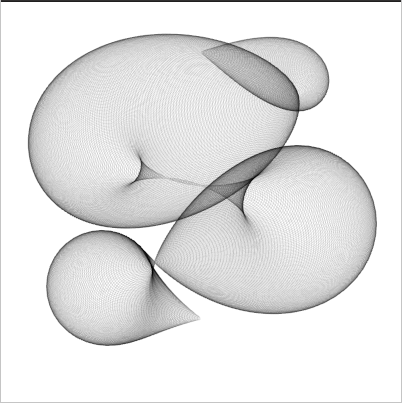## sketch experiment 3 - circles

my third sketch experiment is using a similar technique I used in a previous ronin example - drawing a lot of circles along a curved path with a very transparent pen.

Its still a static image that gets rendered - I din't experiment with animations yet, but its very high on my todolist.``````(make-instance 'blub )
(defsketch blub ()
(background (rgb 1 1 1))
(translate 200 50)
(dotimes (i 720)
(with-pen (make-pen :stroke (rgb 0 0 0 0.1 ))
(push-matrix)

(setf f (* (sin (* 6.28 (/ i 720)) ) (+ 0 (* 100 (cos (/ i 72.3))))))
(circle (* 100 (sin (* 3.14 (/ i 180)))) (/ i 2.7) f)
(pop-matrix )
)
)
)
``````